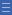# Dividing

Dividing

Fractions express the process of dividing, as well as being the outcome of division (the quotient). Students explore fractions through modelling sharing-as-division problems.

The relationship between fractions and the division process can be described in several ways. Careful attention needs to be given to noticing what constitutes the whole in each situation.

• Partitioning an object into equal parts. This is commonly represented with area diagrams, using shapes such as circles and squares. The shape itself represents one whole.
• Partitioning a group of objects (a collection) into equal parts. The entire group is one whole.
The modelling of fractions using a group of items gives a strong visual connection with whole-number division. Through finding fractions of collections, students realise that, for example, dividing 8 into 2 equal groups is the same as finding half of 8.
Where sharing results in a remainder, the division process can continue by subdividing the remaining parts into pieces less than one. For example, 5 ÷ 2 = 2 remainder 1. The remainder of 1 can be further divided into two equal parts, resulting in 2$$\frac{1}{2}$$.
• Where the collection is smaller than the number of shares to be made, fractions must be formed by partitioning the objects.
For example, 'one cake shared among three people' requires the cake to be partitioned into thirds, so that each person receives one-third: 1 ÷ 3 = $$\frac{1}{3}$$ and so $$\frac{1}{3}$$ = 1 ÷ 3.

The fact that fraction notation itself expresses division emerges from experience with this type of division.

Further information about fractions as division can be found in the article Fractions as Division: The Forgotten Notion? on the AAMT website.

Yes

Yes

Name Class SectionFractions of collections Folder 17Sharing as division Folder 17